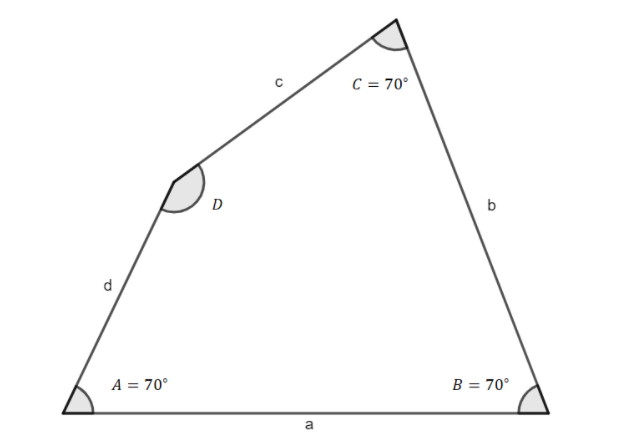Courses
Courses for Kids
Free study material
Offline Centres
MoreLast updated date: 05th Dec 2023
Total views: 280.2k
Views today: 2.80k

# If three acute angles of a quadrilateral measure 70° each, then the measure of the fourth angle is___________________.Verified
280.2k+ views
Hint: Study the property of the quadrilateral. Learn about the measure of the four angles of the quadrilateral. The measure of four angles of a quadrilateral is 360 degrees.
Formula used: The sum of the four angles of a quadrilateral ABCD is, $\angle A + \angle B + \angle C + \angle D = {360^ \circ }$ where, $\angle A,\angle B,\angle C$ and $\angle D$ are the four angles of the quadrilateral.

Complete step by step solution:
We have given here a quadrilateral whose three angles are acute angles. Measure of each angle of the quadrilateral is ${70^ \circ }$ . We have to find the measure of the fourth angle.
Now, we know that the sum of the four angles of a quadrilateral ABCD is, $\angle A + \angle B + \angle C + \angle D = {360^ \circ }$ where, $\angle A,\angle B,\angle C$ and $\angle D$ are the four angles of the quadrilateral.
So, the sum of three angles of the quadrilateral given here is,$\angle A + \angle B + \angle C = {70^ \circ } \times 3 = {210^ \circ }$ [ Let, four angles are $\angle A,\angle B,\angle C$ and $\angle D$ respectively]
So, the fourth angle of the quadrilateral is the difference between 360 and the sum of the three angles. $\angle D = {360^ \circ } - (\angle A + \angle B + \angle C)$
Or, $\angle D = {360^ \circ } - {210^ \circ } = {150^ \circ }$
So, the measure of the fourth angle is ${150^ \circ }$
So, the correct answer is “ ${150^ \circ }$ ”.

Note: The sum of the three angles of a triangle is ${180^ \circ }$ and the sum of the four angles of a quadrilateral is always equal to ${360^ \circ }$ . For different types of quadrilaterals with the said property they have additional properties. For example, the sum of opposite angles of a quadrilateral inside a circle is ${180^ \circ }$ . Each angle of a square is a right angle. The opposite angle of a parallelogram is equal.